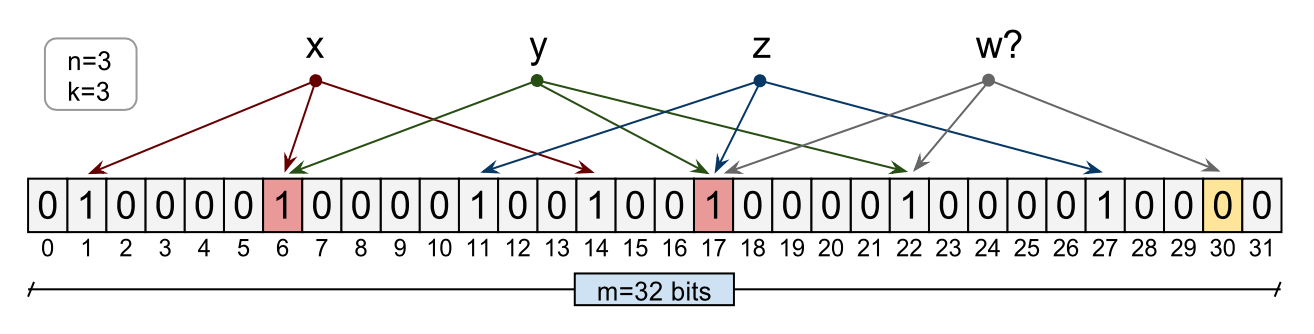# 1133. Data Structure - Bloom FilterBloom Filter

Bloom filter and its implementation.

## 1. Bloom Filter

Bloom filter is a data structure designed to rapidly and memory-efficiently tell whether an element is present in a set.

The cost paid for this efficiency is that a Bloom filter is a probabilistic data structure: it tells us that the element either definitely is not in the set or may be in the set.

The base data structure of a Bloom filter is a Bit Vector.## 2. Implementation

Use an integer array as bit vector. Each cell has the value either 0 or 1. One key will be hashed by three hash functions, then it will be stored into the array.

public class BloomFilter {
private int capacity;
private int[] array;
public BloomFilter(int capacity) {
this.capacity = capacity;
array = new int[capacity];
}

int first = hash_function1(key);
int second = hash_function2(key);
int third = hash_function3(key);
array[first%capacity] = 1;
array[second%capacity] = 1;
array[third%capacity] = 1;
}

public boolean contains(String key) {
int first = hash_function1(key);
int second = hash_function2(key);
int third = hash_function3(key);

int firstIndex = array[first % capacity];
if (firstIndex == 0) {
return false;
}

int secondIndex = array[second % capacity];
if (secondIndex == 0) {
return false;
}

int thirdIndex = array[third % capacity];
if (thirdIndex == 0) {
return false;
}

return true;
}

private int hash_function1(String key) {
int hash = 0;
for (int i = 0; i < key.length(); ++i) {
hash = 33 * hash + key.charAt(i);
}

return Math.abs(hash);
}

private int hash_function2(String key) {
final int p = 16777619;
int hash = (int) 2166136261L;
for (int i = 0; i < key.length(); ++i) {
hash = (hash ^ key.charAt(i)) * p;
}
hash += hash << 13;
hash ^= hash >> 7;
hash += hash << 3;
hash ^= hash >> 17;
hash += hash << 5;
return Math.abs(hash);
}

private int hash_function3(String key) {
int hash, i;
for (hash = 0, i = 0; i < key.length(); ++i) {
hash += key.charAt(i);
hash += (hash << 10);
hash ^= (hash >> 6);
}
hash += hash << 13;
hash ^= hash >> 11;
hash += hash << 15;
return Math.abs(hash);
}
}


## 3. Performance Test

### 3.1 HashSet

We define a hashset with capacity 100,000,000 and insert 10,000,000 entries into the set. Then, we assert several elements to test whether they are in the set.

public class HashSetTest {
@Test
public void testHashSet() {
int capacity = 100000000;
int count = capacity / 10;
long start = System.currentTimeMillis();
Set<Integer> set = new HashSet<>(capacity);
for (int i = 0; i < count; i++) {
}
assertTrue(set.contains(1));
assertTrue(set.contains(2));
assertTrue(set.contains(3));
assertTrue(set.contains(999999));
assertFalse(set.contains(10000001));
assertFalse(set.contains(400230340));

long end = System.currentTimeMillis();
System.out.println("Executed Time: " + (end - start));
}
}


It takes more than 6 seconds to execute the test.

Executed Time: 6442


### 3.2 Bloom Filter

Create a similar test with the Bloom Filter structure we built previously.

class BloomFilterTest {
@Test
public void testBloomFilter() {
int capacity = 100000000;
int count = capacity / 10;
long start = System.currentTimeMillis();
BloomFilter bloomFilter = new BloomFilter(capacity);
for (int i = 0; i < count; i++) {
}
assertTrue(bloomFilter.contains(1 + ""));
assertTrue(bloomFilter.contains(2 + ""));
assertTrue(bloomFilter.contains(3 + ""));
assertTrue(bloomFilter.contains(999999 + ""));
assertFalse(bloomFilter.contains(10000001 + ""));
assertFalse(bloomFilter.contains(400230340 + ""));

long end = System.currentTimeMillis();
System.out.println("Executed Time: " + (end - start));

}
}


It takes only about 1 second to complete the test, which is much faster than the hash set.

Executed Time: 1171